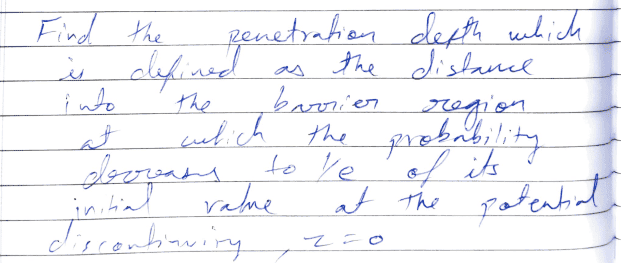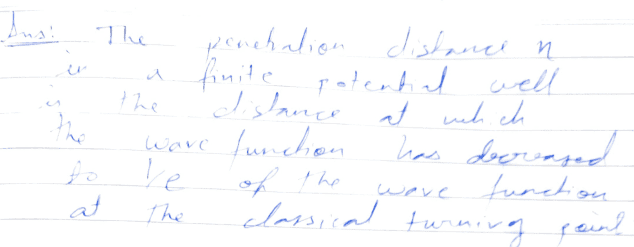# Finite potential well problem penetration depth

Ashish Somwanshi
Homework Statement:
Consider a potential step with a height of V0=0.5eV. An electron is incident from the lower potential side (where the potential energy is set to equal to zero) with an energy E=0.3eV.

Find the penetration depth which is defined as the distance into the barrier region at which the probability decreases to 1/e of its initial value at the potential discontinuity, z=0.

Relevant Equations:
Eta = hket/(2m(Vo-E)), where eta is the penetration depth and hket=1.05×10^(-34)
I don't understand where I went wrong, the formula and calculations which I have attached are correct...please do help if anyone can spot the mistake.

#### Attachments

• finite penetration depth.pdf
1 MB · Views: 20

Homework Helper
Gold Member
If possible, next time please type in your solution rather than post pictures of handwritten solutions.

Regarding your solution, did you take into account the distinction between "probability" and "probability amplitude"?

Ashish Somwanshi
Formula is independent of probability or probability amplitude and autograder says my answer of 0.434nm is incorrect. I have just substituted values given E and Vo and mass of electron and hket in the above formula for penetration depth in case of finite potential well. What has probability and probability amplitude got to do with the question?? You can yourself check and using the formula you will get value of 0.434nm.

#### Attachments

•Screenshot_20221007_220858.jpg
19.7 KB · Views: 14
Homework Helper
Gold Member
Formula is independent of probability or probability amplitude and autograder says my answer of 0.434nm is incorrect. I have just substituted values given E and Vo and mass of electron and hket in the above formula for penetration depth in case of finite potential well. What has probability and probability amplitude got to do with the question?? You can yourself check and using the formula you will get value of 0.434nm.
It is indeed true that if one uses the formula that you provided, one will get the answer you got. This means that you substituted the numbers in the formula correctly. However, what makes you think that the formula $$\eta =\frac{\hbar}{\sqrt{2m(V_0-E)}}$$ gives the length at which the probability drops to ##1/e## of its value at ##z=0##? In your notes you say "It can be derived". Fair enough. Please provide your derivation because I don't believe that the formula is independent from the probability or probability amplitude. The two drop to ##1/e## of their initial values at different distances from the discontinuity. I think you calculated the wrong ##\eta## but without its derivation I cannot be sure. However, it seems that the autograder agrees with me.

Homework Helper
Gold Member
Formula is independent of probability or probability amplitude and autograder says my answer of 0.434nm is incorrect. I have just substituted values given E and Vo and mass of electron and hket in the above formula for penetration depth in case of finite potential well. What has probability and probability amplitude got to do with the question?? You can yourself check and using the formula you will get value of 0.434nm.

You have two different definitions of penetration depth.

First definition:Second definition.The first definition is the distance where the probability is reduced by 1/e. The second definition is the distance where the wave function (also known as the probability amplitude) is reduced by 1/e. Your answer is found using the second definition. Maybe they want the answer based on the first definition.

Homework Helper
Gold Member
The first definition is the distance where the probability is reduced by 1/e. The second definition is the distance where the wave function (also known as the probability amplitude) is reduced by 1/e. Your answer is found using the second definition. Maybe they want the answer based on the first definition.
Yes, the formula used by OP is for the wavefunction, not the probability.

Ashish Somwanshi
It is indeed true that if one uses the formula that you provided, one will get the answer you got. This means that you substituted the numbers in the formula correctly. However, what makes you think that the formula $$\eta =\frac{\hbar}{\sqrt{2m(V_0-E)}}$$ gives the length at which the probability drops to ##1/e## of its value at ##z=0##? In your notes you say "It can be derived". Fair enough. Please provide your derivation because I don't believe that the formula is independent from the probability or probability amplitude. The two drop to ##1/e## of their initial values at different distances from the discontinuity. I think you calculated the wrong ##\eta## but without its derivation I cannot be sure. However, it seems that the autograder agrees with me.
There was no derivation of this formula in my notes. I found this formula from the net. It seems now I have to provide my original notes for further inspection. As soon as I finish writing my complete notes. I will post it here.

Homework Helper
Gold Member
2022 Award
Hi @Ashish Somwanshi. Your notes say that ##\eta## is the distance for the wavefunction (##\psi(z)##) to fall from ##\psi (L)## to ##\frac 1e \psi(L)##. That’s where your equation ##\psi(L+\eta)## = ##\frac 1e \psi(L)## comes from.

But the question is asking you to find “the distance into the barrier region at which the probability decreases to 1/e of its value at z=0”.

This distance is not ##\eta##, but is closely related to it.

##\psi(z)## does not give probability. Do you know how to find probability (or more correctly, probability density) from ##\psi##? It’s one of the most basic things you first learn about wave functions.

Ashish Somwanshi
Just square the absolute value of wavefunction it gives the probability density. So how do I find the penetration depth where the probability density reduces to 1/eof its initial value at z=0. There must be some well knownformula? Is it related to transmission probability?

Homework Helper
Gold Member
Inside the step, the wavefunction behaves as ##\psi(z) = A e^{- z/\eta}## where ##A## is a constant and ##z## is the penetration distance into the step. [Edit: Here, ##\eta = \frac{\hbar}{\sqrt{2m(V_0-E))}}##.]

How does ##|\psi(z)|^2## behave?

Last edited:
Ashish Somwanshi
Inside the step, the wavefunction behaves as ##\psi(z) = A e^{- z/\eta}## where ##A## is a constant and ##z## is the penetration distance into the step.

How does ##|\psi(z)|^2## behave?
Psi^2(z)=A^2*e^(-2z/eta)

Homework Helper
Gold Member
Psi^2(z)=A^2*e^(-2z/eta)
Yes. Can you work with this expression to find the answer?

Homework Helper
Gold Member
There must be some well knownformula? Is it related to transmission probability?
There is no well known formula. You have to derive it yourself. Just think. What is the probability at ##z=0## and at ##z=\eta##? Then write an equation that says that the latter is equal to ##1/e## of the former and solve for ##\eta##.

Homework Helper
Gold Member
@Ashish Somwanshi : In post #11 I was assuming that ##\eta## is the quantity ## \frac{\hbar}{\sqrt{2m(V_0-E))}}##, as given in your handwritten notes. Thus, at ##z = \eta## the wavefunction has decayed to ##\large \frac{1}{e}## of its value at ##z = 0##.

Ashish Somwanshi
I got A^2=(1/e)A^2, now how I am supposed to solve for eta?

Last edited:
Ashish Somwanshi
I am not getting the value of eta from method suggested by @kuruman

Ashish Somwanshi
Isn't this problem already solved at chegg? Can anyone can check it up on chegg and give a hint or method of action?

Homework Helper
Gold Member
If ##\eta = \frac{\hbar}{\sqrt{2m(V_0-E))}}##, then ##\psi(z) = A e^{- z/\eta}## and ##\psi^2(z) = A^2 e^{-2 z/\eta}##.

So, what is ##\psi^2(z)## when ##z = 0##? For what value of ##z## will ##\psi^2(z)## be reduced to 1/e of the value at ##z = 0##?

Ashish Somwanshi
If ##\eta = \frac{\hbar}{\sqrt{2m(V_0-E))}}##, then ##\psi(z) = A e^{- z/\eta}## and ##\psi^2(z) = A^2 e^{-2 z/\eta}##.

So, what is ##\psi^2(z)## when ##z = 0##? For what value of ##z## will ##\psi^2(z)## be reduced to 1/e of the value at ##z = 0##?
Thanks extremely, @kuruman and @TSny , I've passed the course and got the correct answer of 0.217nm for penetration depth for which the probability density reduces to 1/2.71828 of its initial value at z=0 of the potential step.